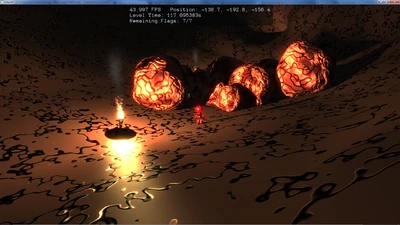## FANDOM

69 Pages

Making a node and adding an particle effect:

```// as with all things in Urho, you don't really need a node just for
// the particle effect but here I needed an offset
Node* n_particle=node->CreateChild();n_particle->Translate(Vector3(0,1.6,0));ParticleEmitter* emitter=n_particle->CreateComponent<ParticleEmitter>();emitter->SetEffect(cache->GetResource<ParticleEffect>("Particle/torch_fire.xml"));
```

You can also add multiple emitters (with different effects for example) to the same node.

It's also possible to modify an particle effect to control stuff like the emitting rate:

```ParticleEffect* effect_exhaust=emitter->GetEffect();
effect_exhaust->SetMinEmissionRate(engine/5);  // here the engine exhaust (smoke)effect_exhaust->SetMaxEmissionRate(engine/5);  // depends on the engine power
```

### Particle ScriptsEdit

Example for a fire effect:

```<?xml version="1.0"?><particleeffect>    <material name="Materials/Particle.xml" />    <numparticles value="1000" />    <updateinvisible enable="true" />    <relative enable="false" />    <scaled enable="true" />    <sorted enable="false" />    <animlodbias value="0" />    <emittertype value="box" />    <emittersize value="0.05 0.05 0.05" />    <direction min="-0.1 0.02 -0.1" max="0.1 0.02 0.1" />    <constantforce value="0 2 0" />    <dampingforce value="1" />    <activetime value="0" />    <inactivetime value="0" />    <emissionrate min="40" max="45" />    <particlesize min="0.1 0.1" max="0.3 0.3" />    <timetolive min="2" max="2" />    <velocity min="0" max="0.5" />    <rotation min="0" max="0" />    <rotationspeed min="0" max="0" />    <sizedelta add="0" mul="0.8" />    <colorfade color="1 1 0.45 0" time="0" />    <colorfade color="1 0.63 0.45 1" time="0.5" />    <colorfade color="0.5 0.32 0.22 0.5" time="1.5" />    <colorfade color="0 0 0 0" time="2" /></particleeffect>
```

This combined with this smoke effect (both emitter are on the same node):

```<?xml version="1.0"?><particleemitter>    <material name="Materials/Smoke.xml" />    <numparticles value="1000" />    <updateinvisible enable="true" />    <relative enable="false" />    <activetime value="0" />    <animlodbias value="0" />    <inactivetime value="0" />    <emissionrate min="20" max="25" />    <sorted enable="false" />    <rotationspeed min="-30" max="30" />    <emittertype value="box" />    <emittersize value="0.05 0.05 0.05" />    <direction min="-0.1 0.02 -0.1" max="0.1 0.02 0.1" />    <dampingforce value="1" />    <velocity min="0" max="0.5" />    <particlesize min="0.1 0.1" max="0.2 0.2" />    <sizedelta add="0" mul="1.5" />    <timetolive value="4" />    <constantforce value="0 2 0" />    <colorfade color="0.2 0.2 0.2 0" time="0.0" />    <colorfade color="0.2 0.2 0.2 0" time="1.5" />    <colorfade color="0.2 0.2 0.2 0.1" time="2.0" />    <colorfade color="0.6 0.6 0.6 0" time="4.0" /></particleemitter>
```

Gives a fire effect (used by the torches in USP):(I have no idea why one is a "particleeffect" and one a "particleemitter". Maybe one is an older, obsolete format which still works.)

In Urho there's some flickering when two emitter are close to each other (like at the same node) and the particles overlap. In USP this was avoided by fading the smoke particles in when the fire particle are already gone / faded out (as you can see at the given script examples).

I don't know if there is documentation about all the properties, but you can see them all in the API documentation (because you can also set everything in code and change it dynamically): http://urho3d.github.io/documentation/1.32/class_urho3_d_1_1_particle_effect.html

Community content is available under CC-BY-SA unless otherwise noted.